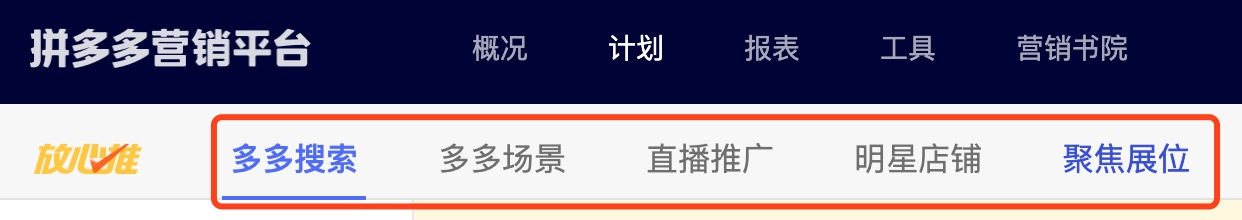Time Flying### 商家端模块职责

• 创建店铺: 官网 -> 商家入驻
• 目前 BApp + 微信的入驻率不容小觑。
• 模块职责
• 发货管理: 处理日常发货、退换货等服务。
• 订单查询
• 发货中心
• 急速发货
• 物流工具
• 物流概况
• 包裹中心
• 电子面单
• 打单工具
• 订单开票
• 正品心智
• 与商家评分打通
• 售后管理
• 售后工作台
• 工单管理
• 商家举证
• 小额打款
• 退货包运费
• 急速退款
• 商品管理
• 发布新商品
• 店铺装修
• 商品列表
• 商品体验
• 商品素材
• 评价管理
• 商品工具
• 供货管理
• 批发供货
• 分销
• 快团团供货
• 采购管理
• 批发采购
• 采购订单
• 店铺营销
• 营销活动
• 竞价活动
• 取倒数第二位价格: 平台与商家的博弈, 商家提前知道竞价规则。这时商家会产生错觉, "我的价格是最低的, 我能盈利比我竞价的价格多一些的利润", 所以在竞价的时候会更接近成本的竞价。case: 多多果园。
• 平台招标
• 营销工具
• 转化提升
• 优惠券
• 限时限量
• 秒杀
• 收藏、满返(部分代替购物车)
• 粉丝运营
• 关注(直播等)
• 3 ~ 5 块一个粉丝
• 群聊(红包群)
• 店铺设砍价
• 短信营销
• 店铺装修
• 店铺页设计
• 多多直播
• 数据中心
• 账户资金
• 多多客服
• 多多进宝
• 推广中心
• 店铺管理
• 商家服务市场
• 多多国际
• 消费者不匹配 —> 海淘价廉物品
• 不敢买 —> 溯源系统、代报关
• 不了解品牌 —> 种草
• 装修
• 店铺装修
• 商详装修
• 品牌装修
• 小程序装修

### 运营引流

• 运营
• 视觉运营
• 店铺视觉
• 品牌型
• 价格型
• 店铺指标
• 店铺转换率
• 热销产品排行
• 首页关注指标
• 访问量、访客量
• 跳失率、出店率
• 首页到商品详情页、分类页点击率
• 口碑运营
• 客服运营
• 引流推广
• 拼多多流量来源
• 社交裂变
• 拼单卡、砍价免费拿、多多果园等引流渠道
• 广告
• CPT(Cost Per Time, 按时长付费)、CPM(Cost Per Mile, 每千人成本)、CPC(Cost Per Click, 按单次点击扣费)、明星店铺推广、数据银行推广、品类关键词库、短信营销等引流渠道
• App 展示
• 首页开屏、首页 Banner、类目类 Banner、个人中心 Banner、新人专区、App 推送消息等引流渠道
• 搜索
• 活动
• 限时秒杀、品牌清仓、名片折扣、爱逛街、食品超市、时尚穿搭、新品推荐、微信公众号推文、微信公众消息和领券中心等引流渠道
• 其它渠道
• 多多进宝、微信群等
• 站内免费引流
• 运用标签精准引流
• 千人前面推荐算法 => 人群标签匹配商品标签
• 人群标签
• 用户行为
• 个人属性
• 用户喜好
• 商品标签
• 类目标签
• 价格标签
• 属性标签
• 站外免费引流
• 付费引流
•• 搜索推广
• 场景展示推广
• 排名公式: 综合排名 = 商品质量分 * 广告单击出价
• 其他推广工具
• 活动引流
• 活动报名
• 营销活动
• 品牌特卖、电器城、爱逛街、9 块 9 特卖、限时秒杀
• 店铺活动
• 限量促销、拼单返现、限时免单
• 竞价活动
• 竞价活动
• 品质招标
• 社交活动
• 助力享免单
• 多多果园
• 推手引流
• 多多进宝
• 综合流量 GMV = 多多进宝 + 自然搜索 + 场景推广